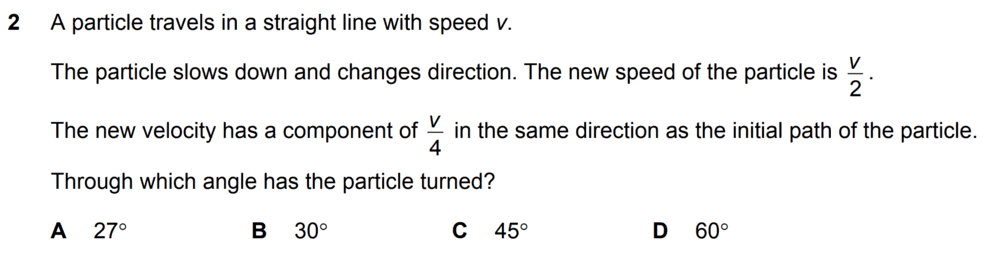• ianc1339
In summary, the conversation discusses resolving vectors into components, whether they always form right angles, and the difference between resolving a vector and finding its components in a given direction. It is possible to resolve a vector into any two directions, but when finding components in non-orthogonal directions, they cannot be added together to recover the original vector.

#### ianc1339

Homework Statement
Posted in image below
Relevant Equations
Basic trigonometric identitiesThe answer is D (60 degrees) and I understand how to get that answer. But this assumes that the new velocity's component of v/4 can form right angles with another component of the new velocity.

So I'm confused whether vector components always form right angles to each other. When I searched this up, I have seen vector diagrams with components that do not form right angles to each other.

ianc1339 said:
Homework Statement:: Posted in image below
Relevant Equations:: Basic trigonometric identities

View attachment 272531

The answer is D (60 degrees) and I understand how to get that answer. But this assumes that the new velocity's component of v/4 can form right angles with another component of the new velocity.

So I'm confused whether vector components always form right angles to each other. When I searched this up, I have seen vector diagrams with components that do not form right angles to each other.
Typically one resolves into components which are at right angles to each other, but it is possible to resolve into any two directions that are not collinear.
Suppose we wish to express vector v in terms of vectors v1, v2. I.e. we need to solve ##\vec v=a_1\vec v_1+a_2\vec v_2##. Taking dot products, ##\vec v.\vec v_1=a_1\vec v_1^2+a_2\vec v_1.\vec v_2## and, ##\vec v.\vec v_2=a_1\vec v_1.\vec v_2+a_2\vec v_2^2##. That's a pair of simultaneous equations we can solve for a1, a2.
But if we ask what components a vector v has in the two directions, that's different. The component of v in the direction v1 is ##c_1\vec v_1=\frac {\vec v.\vec v_1} {|\vec v_1|^2}\vec v_1##.
If v1 and v2 above are at right angles then ##c_1=a_1##, but not otherwise. That is, if you find the components of a vector in the directions of two vectors not at right angles then you cannot add the components together to recover the original vector.

•ianc1339
Vectors can always be written as the sum of two (or more) component vectors. One does this to make the physics clearer or the math easier to deal with. A useful usual decomposition is to take the component vectors parallel to your choice of coordinate axes. Then the components will always be orthogonal. But the choice is yours...

•ianc1339
haruspex said:
Typically one resolves into components which are at right angles to each other, but it is possible to resolve into any two directions that are not collinear.
Suppose we wish to express vector v in terms of vectors v1, v2. I.e. we need to solve ##\vec v=a_1\vec v_1+a_2\vec v_2##. Taking dot products, ##\vec v.\vec v_1=a_1\vec v_1^2+a_2\vec v_1.\vec v_2## and, ##\vec v.\vec v_2=a_1\vec v_1.\vec v_2+a_2\vec v_2^2##. That's a pair of simultaneous equations we can solve for a1, a2.
But if we ask what components a vector v has in the two directions, that's different. The component of v in the direction v1 is ##c_1\vec v_1=\frac {\vec v.\vec v_1} {|\vec v_1|^2}\vec v_1##.
If v1 and v2 above are at right angles then ##c_1=a_1##, but not otherwise. That is, if you find the components of a vector in the directions of two vectors not at right angles then you cannot add the components together to recover the original vector.

"but it is possible to resolve into any two directions that are not collinear"

"if you find the components of a vector in the directions of two vectors not at right angles then you cannot add the components together to recover the original vector"

You can determine the component in any other direction using a unit vector in that direction. But, when you want to determine the equation for the speed using the other component (resolved in this way), it is not as easy to express the speed as when the normal direction is used.

ianc1339 said:
"but it is possible to resolve into any two directions that are not collinear"

"if you find the components of a vector in the directions of two vectors not at right angles then you cannot add the components together to recover the original vector"